# models – astronomy-specific models for pymodelfit¶

This module makes use of the pymodelfit package to define a number of models inteded for data-fitting that are astronomy-specific. Note that pymodelfit was originally written as part of astropysics, and hence it is tightly integrated (including the Fit GUI, where relevant) to other parts of astropysics, often using the models in this module.

Note

Everything in the pymodelfit package is imported to this package. This is rather bad form, but is currently done because other parts of astropysics is written for when pymodelfit was part of astropysics. This may change in the future, though, so it is recommended that user code always use from pymodelfit import ... instead of from astropysics.models import ....

## Classes and Inheritance Structure¶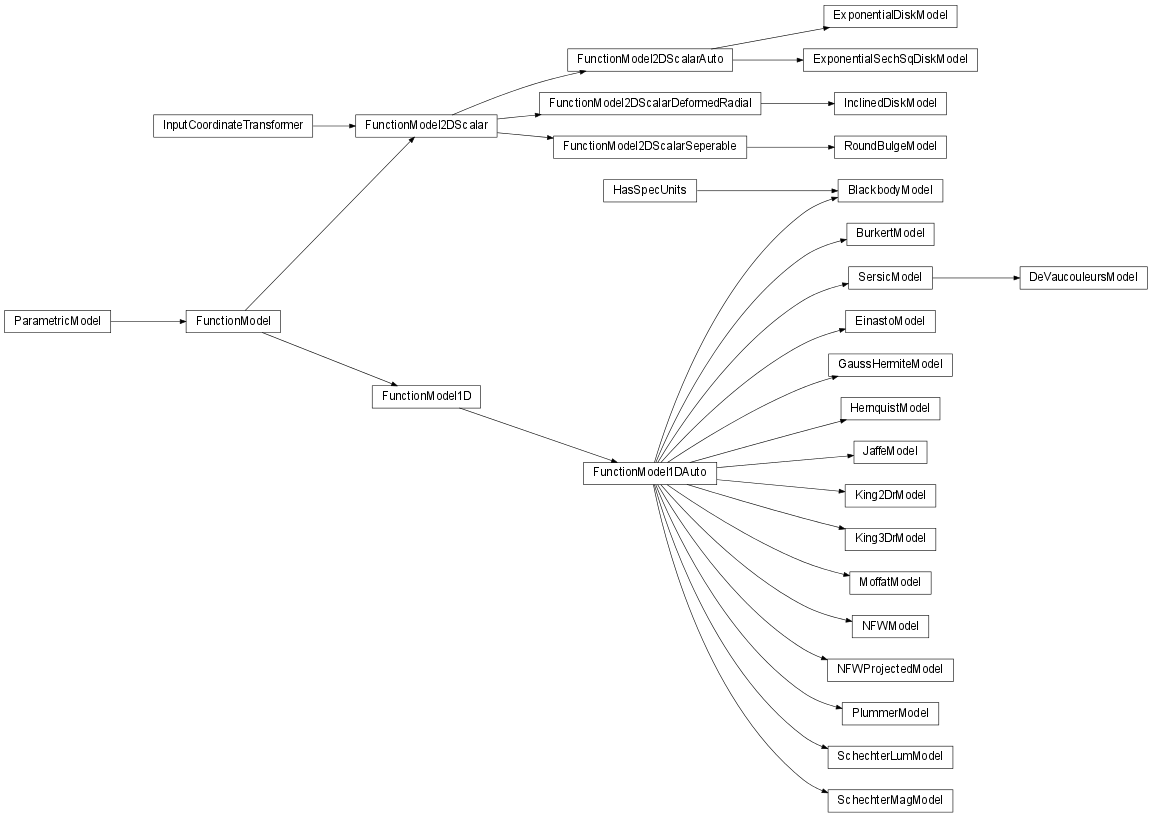## Module API¶

class astropysics.models.BlackbodyModel(unit='wl')

Output/y-axis is taken to to be specific intensity.

A = 1
T = 5800
c = 29979245800.0
f(x, A=1, T=5800)

Returns the flux density at the requested wavelength for a blackbody of the given radius at a specified distance.

Parameters: x (float) – x-value of the model radius (float) – radius of the blackbody in cm distance (float) – distance to the blackbody in cm flux/wl at the specified distance from the blackbody

Determines the distance to a spherical blackbody of the specified radius assuming the flux is given by the model at the given temperature.

Determines the radius of a spherical blackbody at the specified distance assuming the flux is given by the model at the given temperature.

h = 6.626068e-27
kb = 1.3807e-16
peak
rangehint

Sets A so that the output is the flux at the specified distance from a spherical blackbody with the specified radius.

Parameters: radius (float) – Radius of the blackbody in cm distance (float) – distance to the blackbody in cm
setIntensity()

Sets A so that the output is specific intensity/surface brightness.

static stephanBoltzmannLaw(T, area=1)

assumes cgs units

wienDisplacementLaw(peakval)

Uses the Wien Displacement Law to calculate the temperature given a peak input location (wavelength, frequency, etc) or compute the peak location given the current temperature.

Parameters: peakval (float or None) – The peak value of the model to use to compute the new temperature. The temperature for the provided peak location or the peak location for the current temperature if peakval is None.
xaxisname
yaxisname = 'I'
class astropysics.models.BurkertModel

Burkert (1996) profile defined as: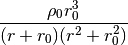where rho0 is the central density.

f(r, rho0=1, r0=1)
r0 = 1
rangehint
rho0 = 1
xaxisname = 'r'
yaxisname = 'rho'
class astropysics.models.DeVaucouleursModel

Sersic model with n=4.

Ae = 1
f(r, Ae=1, re=1)
re = 1
xaxisname = 'r'
class astropysics.models.EinastoModel

Einasto profile given by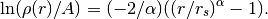A is the density where the log-slope is -2, and rs is the corresponding radius. The alpha parameter defaults to .17 as suggested by Navarro et al. 2010

Note

The Einasto profile is is mathematically identical to the Sersic profile (SersicModel), although the parameterization is different. By Convention, Sersic generally refers to a 2D surface brightness/surface density profile, while Einasto is usually treated as a 3D density profile.

A = 1
alpha = 0.17
f(r, A=1, rs=1, alpha=0.17)
rs = 1
xaxisname = 'r'
yaxisname = 'rho'
class astropysics.models.ExponentialDiskModel

A disk with an exponential profile along both vertical and horizontal axes. The first coordinate is the horizontal/in-disk coordinate (scaled by l) wile the second is z. i.e.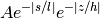for pa = 0 ; non-0 pa rotates the profile counter-clockwise by pa radians.

A = 1
f(inarr, A=1, l=2, h=1, pa=0)
h = 1
l = 2
pa = 0
rangehint
class astropysics.models.ExponentialSechSqDiskModel

A disk that is exponential along the horizontal/in-disk (first) coordinate, and follows a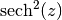profile along the vertical (second) coordinate. i.e.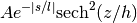for pa = 0 ; non-0 pa rotates the profile counter-clockwise by pa radians.

A = 1
f(inarr, A=1, l=2, h=1, pa=0)
h = 1
l = 2
pa = 0
rangehint
class astropysics.models.GaussHermiteModel

Model notation adapted from van der Marel et al 94

hj3 are h3,h4,h5,... (e.g. N=len(hj3)+2 )

A = 1
f(v, A=1, v0=0, sig=1, *hj3)
gaussHermiteMoment(l, f=None, lower=-inf, upper=inf)

compute the requested moment on the supplied function, or this object if f is None

paramnames = 'h'
paramoffsets = 3
rangehint
sig = 1
v0 = 0
class astropysics.models.HernquistModel

Hernquist (1990) profile defined as: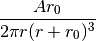where A is the total mass enclosed. Note that r0 does not enclose half the mass - the radius enclosing half the mass is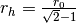.

Note

This form is equivalent to an AlphaBetaGammaModel with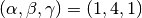, but with a slightly different overall normalization.

A = 1
f(r, A=1, r0=1)
r0 = 1
rangehint
xaxisname = 'r'
yaxisname = 'rho'
class astropysics.models.InclinedDiskModel(inc=0, pa=0, degrees=True, **kwargs)

Inclined Disk model – identical to FunctionModel2DScalarDeformedRadial but with inclination (inc and incdeg) and position angle (pa and padeg) in place of axis-ratios.

class astropysics.models.JaffeModel

Jaffe (1983) profile as defined in Binney & Tremaine as: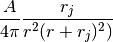where A is the total mass enclosed. rj is the radius that encloses half the mass.

Note

This form is equivalent to an AlphaBetaGammaModel with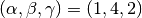, but with a slightly different overall normalization.

A = 1
f(r, A=1, rj=1)
rangehint
rj = 1
xaxisname = 'r'
yaxisname = 'rho'
class astropysics.models.King2DrModel

2D (projected/surface brightness) King model of the form: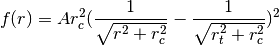King (1966) AJ Vol. 71, p. 64

A = 1
f(r, rc=1, rt=2, A=1)
rangehint
rc = 1
rt = 2
xaxisname = 'r'
class astropysics.models.King3DrModel

3D (deprojected) King model of the form: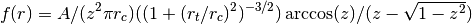A = 1
f(r, rc=1, rt=2, A=1)
rangehint
rc = 1
rt = 2
xaxisname = 'r'
class astropysics.models.MoffatModel

Moffat function given by: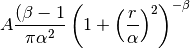A = 1
FWHM

Full Width at Half Maximum for this model - setting changes alpha for fixed beta

alpha = 1
beta = 4.765
f(r, A=1, alpha=1, beta=4.765)
rangehint
class astropysics.models.NFWModel

A Navarro, Frenk, and White 1996 profile – the canonical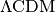dark matter halo profile: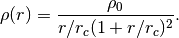Where unspecified, units are r in kpc and rho in Msun pc^-3, although units can always be arbitrarily scaled by rescaling rho0.

Note

This form is equivalent to an AlphaBetaGammaModel with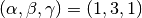, but with a slightly different overall normalization. This class also has a number of helper methods.

static Cvir_to_Mvir(Cvir, z=0)

from Maller & Bullock ‘04 M_sun

Warning

These scalings are approximations.

Parameters: Cvir (float) – Concentration parameter rvir/rc z (float) – Redshift Virial mass in Msun
static Mvir_to_Cvir(Mvir, z=0)

from Maller & Bullock ‘04 M_sun

Warning

These scalings are approximations.

Parameters: Mvir (float) – Virial Mass in Msun z (float) – Redshift Concentration parameter (rvir/rc)
static Mvir_to_Rvir(Mvir, z=0)

from Bullock ‘01 M_sun,kpc

Warning

These scalings are approximations.

Parameters: Mvir (float) – Virial Mass in Msun z (float) – Redshift Virial radius in kpc
static Mvir_to_Vmax(Mvir, z=0)

Convert virial mass to vmax using the scalings here

Warning

These scalings are approximations.

Parameters: Mvir (float) – Virial Mass in Msun z (float) – Redshift Maximum circular velocity in km/s
static Mvir_to_Vvir(Mvir, z=0)

from Bullock ‘01 km/s,M_sun

Warning

These scalings are approximations.

Parameters: Mvir (float) – Virial Mass in Msun z (float) – Redshift Virial Velocity in km/s
static RvirMvir_to_Vvir(Rvir, Mvir)

Computes virial velocity from virial radius and virial mass.

Parameters: Rvir (float) – Virial Radius in kpc Mvir (float) – Virial Mass in Msun Virial Velocity in km/s
static Rvir_to_Mvir(Rvir, z=0)

from Bullock ‘01 M_sun,kpc

Warning

These scalings are approximations.

Parameters: Rvir (float) – Virial Radius in kpc z (float) – Redshift Virial mass in Msun
static Vmax_to_Mvir(Vmax, z=0)

Convert vmax to virial mass using the scalings here

Warning

These scalings are approximations.

Parameters: Vmax (float) – Maximum circular velocity in km/s z (float) – Redshift Virial mass in Msun
static Vmax_to_Rvir(Vmax, z=0)

Convert vmax to virial radius using the scalings here.

Warning

These scalings are approximations.

Parameters: Vmax (float) – Maximum circular velocity in km/s z (float) – Redshift Virial radius in kpc
static Vmax_to_Vvir(Vmax)

from Maller & Bullock ‘04 good from 80-1200 km/s in vvir

Warning

These scalings are approximations.

Parameters: Vmax (float) – Maximum circular Velocity in km/s z (float) – Redshift Virial Velocity in km/s
static Vvir_to_Mvir(Vvir, z=0)

from Bullock ‘01 km/s,M_sun

Warning

These scalings are approximations.

Parameters: Vvir (float) – Virial velocity in km/s z (float) – Redshift Virial mass in Msun
static Vvir_to_Tvir(Vvir, mu=0.59)

Compute the Virial temperature for a given virial radius.

From Barkana & Loeb 2001: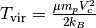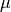is the mean molecular weight, which is 0.59 for fully ionized primordial gas, .61 for fully ionized hydrogen but singly ionized helium, and 1.22 neutral.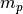is the proton mass,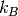is the Boltzmann constant, and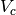is the virial velocity.

Parameters: Vvir (float) – Virial velocity in km/s mu (float) – Mean molecular weight Virial Temperatur in Kelvin
static Vvir_to_Vmax(Vvir)

from Bullock ‘01 good from 80-1200 km/s in vvir

Warning

These scalings are approximations.

Parameters: Vvir (float) – Virial velocity in km/s Virial velocity in km/s
static create_Cvir(Cvir, z=0)

Generates a new NFWModel with the given Mvir using the Cvir_to_Mvir() function to get the scaling.

Warning

These scalings are approximations.

Parameters: Cvir (float) – Concentration parameter (rvir/rc) z (float) – Redshift at which the model is valid A NFWModel object with the provided Cvir and normalization set by the approximate scalings here.
static create_Mvir(Mvir, z=0)

Generates a new NFWModel with the given Mvir using the Mvir_to_Cvir() function to get the scaling.

Warning

These scalings are approximations.

Parameters: Mvir (float) – Virial Mass in Msun z (float) – Redshift at which model is valid A NFWModel object with the provided Mvir and concentration set by the approximate scalings here.
static create_Rvir(Rvir, z=0)

Generates a new NFWModel with the given Mvir using the Mvir_to_Cvir and Rvir_to_Mvir() functions to get the scaling.

Warning

These scalings are approximations.

Parameters: Rvir (float) – Virial Radius in kpc z (float) – Redshift at which the model is valid A NFWModel object with the provided Rvir and concentration set by the approximate scalings here.
static create_VmaxRvmax(vmax, rvmax)

Generates a new NFWModel with the given Vmax and R_Vmax.

Parameters: vmax (float) – maximum circular velocity in km/s. rvmax (float) – radius of maximum circular velocity in kpc.

See Bullock et al. 2001 for reflated reference.

Returns: A NFWModel object matching the supplied vmax and rvmax
deltavir(z=0)

Returns the virial overdensity at a given redshift.

This method should be overridden if a particular definition of virial radius is desired. E.g. to use r200 instead of rvir, do:

nfwmodel.deltavir = lambda z:200

Parameters: z (scalar) – redshift at which to compute virial overdensity virial overdensity
f(r, rho0=1, rc=1)
getC(z=0)

Returns the concentration of this profile(rvir/rc) for a given redshift.

Note

If setC() has been called with an explicitly provided Rvir and Mvir, the concentration will be saved and this will return the saved concentration instead of one computed for a given redshift. If this is not desired, delete the _c attribute after calling setC() .

getMv(z=0)

Gets the mass within the virial radius (for a particular redshift)

getRhoMean(r)

Computes the mean density within the requested radius, i.e.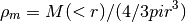density in units of Msun pc^-3 assuming r in kpc

getRv(z=0)

Get the virial radius at a given redshift, with deltavir choosing the type of virial radius - if ‘fromcosmology’ it will be computed from the cosmology, otherwise, it should be a multiple of the critical density

units in kpc for mass in Msun

getV(r, **kwargs)

Computes the circular velocity of the halo at a given radius. i.e.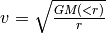.

Parameters: r – The radius (or radii) at which to compute the circular velocity, in kpc.

Additional keyword arguments are passed into integrateSpherical().

Returns: The circular velocity at r (if r is a scalar, this will return a scalar, otherwise an array)
getVmax()

Computes the maximum circular velocity of this profile.

Returns: (vmax,rvmax) where vmax is the maximum circular velocity in km/s and rvmax is the radius at which the circular velocity is maximized.
integrateSpherical(lower, upper, method=None, **kwargs)

NFW Has an analytic form for the spherical integral - if the lower is not 0 or or if method is not None, this function will fall back to the numerical version.

rangehint
rc = 1
rho0 = 1
setC(c, Rvir=None, Mvir=None, z=0)

Sets the model parameters to match a given concentration given virial radius and mass.

Parameters: c (scalar) – concentration = rvir/rc Rvir (scalar or None) – virial radius to assume in kpc - if None, will be inferred from Mvir, c and the virial overdensity (see deltavir). Mvir (scalar or None) – virial mass to assume in Msun - if None, will be inferred from Rvir, c, and the virial overdensity (see deltavir), or if Rvir is None, from the current virial mass. z (scalar) – redshift at which to compute virial overdensity if Rvir or Mvir are None. If both are given, this is ignored.
toAlphaBetaGamma()

returns a new AlphaBetaGammaModel based on this model’s parameters.

xaxisname = 'r'
yaxisname = 'rho'
class astropysics.models.NFWProjectedModel
f(R, rc=1, sig0=1)
integrateCircular(lower, upper, method=None, **kwargs)

NFW Has an analytic form for the spherical integral - if the lower is not 0 or or if the keyword ‘numerical’ is True, this function will fall back to FunctionModel1D.integrateCircular

rangehint
rc = 1
sig0 = 1
class astropysics.models.PlummerModel

Plummer model of the form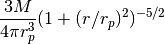M = 1.0
f(r, rp=1.0, M=1.0)
rangehint
rp = 1.0
xaxisname = 'r'
class astropysics.models.RoundBulgeModel(Ae=1, re=1, n=4)

A bulge modeled as a radially symmetric sersic profile (by default the sersic index is kept fixed - to remove this behavior, set the fixedpars attribute to None)

By default, the ‘n’ parameter does not vary when fitting.

fixedpars = ('n',)
class astropysics.models.SchechterLumModel

The Schechter Function, commonly used to fit the luminosity function of galaxies, in luminosity form: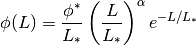SchechterMagModel, Schechter 1976, ApJ 203, 297

Lstar = 10000000000.0
alpha = -1.0
derivative(L, dx=None)

Compute Schechter derivative. if dx is not None, will fallback on the numerical version.

f(L, Lstar=10000000000.0, alpha=-1.0, phistar=1.0)
integrate(lower, upper, method=None, **kwargs)

Analytically Compute Schechter integral using incomplete gamma functions. If method is not None, numerical integration will be used. The gamma functions break down for alpha<=-1, so numerical is used if that is the case.

phistar = 1.0
rangehint
xaxisname = 'L'
yaxisname = 'phi'
class astropysics.models.SchechterMagModel

The Schechter Function, commonly used to fit the luminosity function of galaxies, in magnitude form: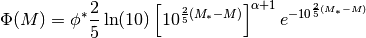SchechterLumModel, Schechter 1976, ApJ 203, 297

Mstar = -20.2
alpha = -1
derivative(M, dx=None)

Compute Schechter derivative for magnitude form. if dx is not None, will fallback on the numerical version.

f(M, Mstar=-20.2, alpha=-1, phistar=1.0)
integrate(lower, upper, method=None, **kwargs)

Analytically compute Schechter integral for magnitude form using incomplete gamma functions. If method is not None, numerical integration will be used. The gamma functions break down for alpha<=-1, so numerical is used if that is the case.

phistar = 1.0
rangehint
xaxisname = 'M'
yaxisname = 'phi'
class astropysics.models.SersicModel

Sersic surface brightness profile: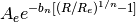Ae is the value at the effective radius re

Note

The Sersic profile is is mathematically identical to the Einasto profile (EinastoModel), although the parameterization is different. By Convention, Sersic generally refers to a 2D surface brightness/surface density profile, while Einasto is usually treated as a 3D density profile.

A0

value at r=0

Ae = 1
static bn_estimate(n)

bn is used to get the half-light radius. If n is None, the current n parameter will be used

The form is a fit from MacArthur, Courteau, and Holtzman 2003 and is claimed to be good to ~O(10^-5)

static bn_exact(n)

Computes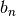exactly for the current sersic index, using incomplete gamma functions.

static exactBn(val=None)

Sets whether the exact Bn calculation is used, or the estimate (see getBn()).

Parameters: val – If None, nothing will be set, otherwise, if True, the exact Bn computation will be used, or if False, the estimate will be used. True if the exact computation is being used, False if the estimate.
f(r, Ae=1, re=1, n=4)
getBn()

Computesfor the current sersic index. If the SersicModel.exactbn attribute is True, this is calculated using incomplete gamma functions (bn_exact()), otherwise, it is estimated based on MacArthur, Courteau, and Holtzman 2003 (bn_estimate()).

n = 4
rangehint
re = 1
sbfit(r, sb, zpt=0, **kwargs)

fit surface brightness using the SersicModel

r is the radial value,sb is surface brightness, zpt is the zero point of the magnitude scale, and kwargs go into fitdata

sbplot(lower=None, upper=None, data=None, n=100, zpt=0, clf=True)

plots the surface brightness for this flux-based SersicModel. arguments are like fitDat

xaxisname = 'r'